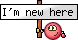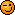View Single Post
SelyJoin Date: Apr 2020
Location: France
Posts: 1
Hi everyone,
I've passed my stage 2 assessments few months ago.
For the maths part : calculator aren't allowed, 20 questions in 30 minutes. The questions include speed-distance-time problems, speed-distance-time with fuel calculation ( fuel used = rate x time ), Pythagoras's theorem, heading (360° a full circle).
Be careful with the units (km/h, miles per hour, miles per minute, ...) and 1,5 hours = 1 hour and 30 min, don't forget to convert the time during calculations.
Check your answers if it makes sense with the question, and check the unit, for example : 45 lbs/min and not 45 min/lbs.
Some informations are given during the assessment, as speed-distance-time formula; 5 miles = 8 km; and 2,2 lbs = 1 kg.
For the physics part : calculator aren't allowed, 20 questions in 30 minutes. The questions include balancing weights, current and resistors, energy, and other topics but I don't really remember.
A paper with formulas are given : Current (amps) = Voltage (volts) / Resistance (ohms); weight = mass x gravity (gravity = 9,81 m/s^2); and other formulas.

I hope it helps you, Good luckPS : Stay calm during the assessments. And if you failed at a test, don't give up, you can do it again!!